Next: A.1 Transverse Field Up: G.D. Morris' Ph.D. Thesis Previous: References

# A Muonium Spin Polarization Function

In this Appendix we derive from first principles the eigenvalues and states of muonium, in the simplest case of free muonium in vacuum with a static magnetic field applied. The polarization functions with the applied magnetic field oriented either transverse to or along the initial muon spin are calculated. Spin relaxation is not considered in this calculation.

In the situation being considered the Hamiltionian of the muonium atom,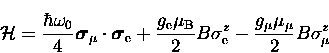(1)
includes the Zeeman terms for the muon and electron spins, and the interaction between these spins.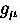= 2.002331846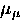=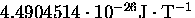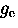= 2.002319304386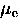=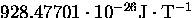In order to determine the time dependence of the muon spin polarization we diagonalize this Hamiltonian and calculate the elements of the muon spin operator. In doing so we will obtain the muonium states and corresponding energies, which determine the precession frequencies observed in the muon spin polarization signal.

We start by defining a set of states of the muonium atom in terms of the individual electron and muon states,

the superscripts on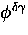referring to the muon and electron spins projected onto the quantization axis, which we take to be the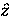direction.

The spin operator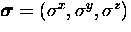has components given by the usual Pauli matrices and the muon and electron spin operators act only on their corresponding parts of the muonium wave function.

The operator in the first term of the Hamiltonian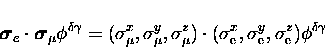(2)
can be written in terms of raising and lowering operators which are defined by

by expanding its first two terms into

and similarly

The third term follows trivially from the definition of the spin along the quantization axis for both the muon and electron,

The hyperfine interaction term is then the sum of Eqs. (A.5,A.6,A.7) and we obtain

Finally, noting that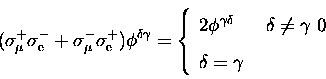(3)
Eq. (A.8) simplifies to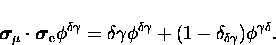(4)

For the two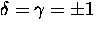cases it then immediately follows that the eigenvalues are given by

and

where we have defined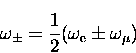(5)
with

In order to diagonalize the Hamiltonian in the other two cases where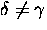we define linear combinations of the basis vectors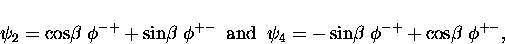(6)
where the parameter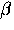turns out to depend on the magnitude of the field B, as will be shown below.

Operating with the full Hamiltonian on the first of these, we obtain

If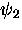is to be an eigenfunction of the Hamiltonian we must have both

where C is an arbitrary constant and we have defined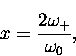(7)
from which it follows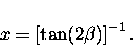(8)

Equation (A.18) can now be simplified to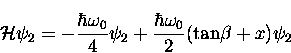(9)
and by using the trigonmetric identity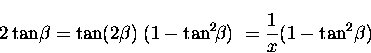(10)
to substitute

we obtain the final result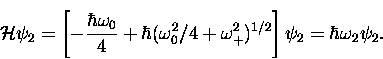(11)

A similar calculation, operating on the wavefunction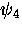, yields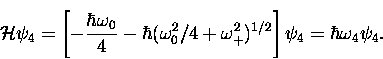(12)

The results so far are summarized in Figure  A.32, which shows the four eigenvalues as functions of the magnetic field B.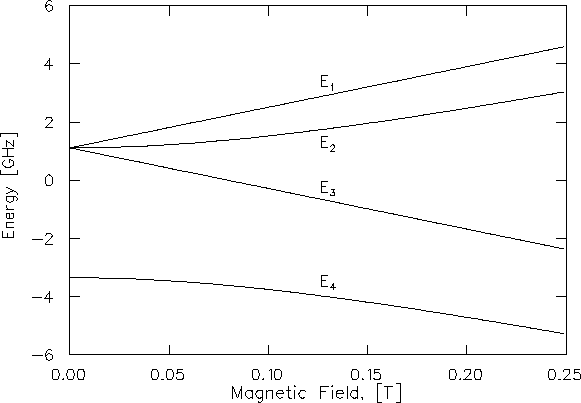We shall see that the polarization signal has components at frequencies determined by the transition energies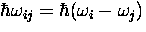between these states. These frequencies areNext: A.1 Transverse Field Up: G.D. Morris' Ph.D. Thesis Previous: References